# Numb3rs Season 4 Episode 13: Black Swan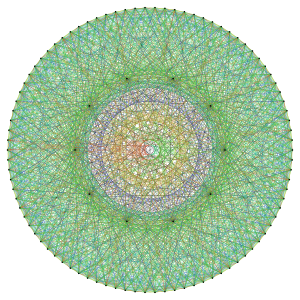In this episode, Charlie suggests using the Floyd-Warshall Algorithm to find a bombing suspect's stash house.

## Graphs

In math, the term "graph" doesn't only mean the plot of functions against x, y, and z axes. It can also refer to a way of representing objects and relationships between these objects. Objects are represented with points, also called "vertices". We draw a line between two vertices to represent any type of connection that we are interested in. These lines are called "edges". The particular way that these points and lines are drawn on the plane is irrelevant. The graph is the logical structure of how these things are related. Abstractly, a graph is nothing more than a list of names for the vertices along with a list of ordered pairs of these same names to represent the edges.

The following is a visual representation of some graph: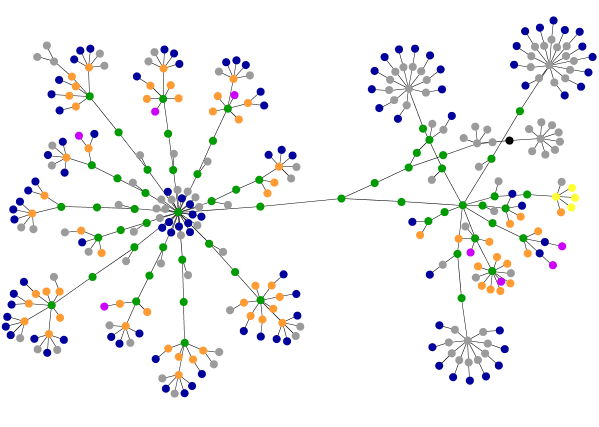In some contexts, graphs are allowed to have multiple edges between the same vertices, or edges from a vertex to itself. Whether such constructions are meaningful depends on how the graph is being used. Edges that connect one vertex to itself are called "loops".

### Directed Graphs

Another type of graph is a "directed graph", where the edges have an orientation, and we think of them as going from one vertex to another vertex. One application for such a construction might be travel in New York City. We represent street intersections in the city by vertices, and we draw an edge from one vertex to another when we can drive from one intersection to the next without traveling through any other intersection. Here, it becomes obvious why the edges need to be directed. When there are one-way streets, we have a directed edge in the direction of the traffic flow.

A path is a sequence of edges where the tail of one edge is the same vertex as the head of the next edge in the sequence. A path in our example graph of New York City's streets corresponds directly to ways to navigate the streets in a car.

### Weighted Graphs

Another modification we can make to our original definition of a graph is to give weights to the edges in our graph. Usually, we restrict the weights to be non-negative. For instance, in our example of the graph of the streets of New York City, we can attach a distance from one intersection to another adjacent intersection. Then, the sum of the weights in a path on the graph corresponds to the length of the corresponding route in the city. Both directed and undirected graphs can have weighted edges. In the former case, such graphs are called "directed weighted graphs".

### Representing Graphs as Matrices

A finite directed weighted graph can be represented by a matrix of values such as: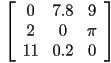The row that an element is in corresponds to the tail of a directed edge (or where the edge is coming from), and the column corresponds to the head of the directed edge (or where the edge is going). The reason for this seemingly arbitrary choice will be explained below. Here, the 7.8 in the first column and second row corresponds to the weight of an edge from the first vertex to the second vertex. The zeroes along the diagonal correspond to a length of zero from a vertex to itself.

One advantage of using matrices to represent graphs is their compactness in representing the information. However, more powerful, is the ability to use matrix arithmetic to answer questions about the structure of the graph. For instance, in a 3-vertex directed graph, we will represent an edge from one vertex to another with a 1 and the lack of an edge with a zero. Such a matrix is called a "transition matrix", because it describes how one can move around the graph while only following edges in their preferred direction.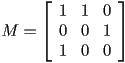This matrix represents a graph with three vertices, where there is a loop at the first vertex (as represented by the 1 at the first location along the diagonal) and where there is an edge from the first vertex to the second, another from the second vertex to the third, and finally from the third vertex back to the first.

Let us multiply this matrix by itself, and look at its square: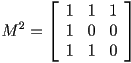The square of the matrix has a nonzero entry whenever you can go from the vertex represented by the row to the vertex represented by the column in exactly two steps. The seemingly arbitrary choice of going from the vertex represented by the row to the one represented by the column is chosen to correspond to an equally arbitrary choice in how we multiply matrices.

The same pattern continues. The cube of the original matrix will have nonzero entries precisely between those edges which are exactly three steps apart and no more or less.

### Activity 1

In general such powers of transition matrices will no longer only have zeroes and ones as entries. However, what the entries actually correspond to is far more interesting. Can you use the definition of matrix multiplication to figure out what is being counted?

## Shortest Path

The shortest path between two nodes is the path with the minimum sum of weights of the edges making up the path. On an unweighted graph, the edges are considered to all have an equal weight of 1, so that the shortest path between two nodes is the path which has the smallest number of edges. Sometimes there is more than one shortest path, if there is a tie. For large graphs, it can be very time-consuming to find the shortest path between two vertices, because, naively you have to check every single possible path between the two vertices.

## Floyd-Warshall

The Floyd-Warshall Algorithm is a technique for finding the shortest path distance between all pairs of vertices simultaneously. Algorithms like this which solve a huge number of related questions simultaneously are pervasive in the computer science and typically fall into a subfield called dynamic programming. What makes these algorithms work so well is that the questions can be split up into smaller sub-problems that many (but perhaps not all) of the original questions share. Here, as will be described shortly, the subproblems consist of finding the shortest paths between vertices that only traverse the first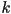vertices. Asincreases, the algorithm will slowly refine its estimates of the shortest path length between vertices, because it will allow more vertices in the candidate paths.

### The Algorithm

Letbe the number of vertices in our graph. We create an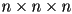matrix of values called, where we initialize the value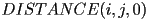to be the weight of the directed edge from vertexto vertex, if such an edge exists, or to infinity if such an edge does not exist.

We interpretto be the minimum length of every path fromtothat has only the vertices fromtoas intermediate steps. We interpretto be the length of paths that have no intermediate steps. After the initialization, the matrixtrivially contains the shortest path length between every pair of vertices, so long as the path contains no other vertices besides the beginning vertex and the ending vertex.

Now, for every pair of verticesandwith a fixed, assume thatcontains the shortest path length betweenandso long as the path contains no vertices besides those fromto. We will show how to compute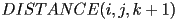for every pair of verticesand.

For any particular, there are two possibilities. Either the shortest path fromtoin the firstvertices goes through vertexor it doesn't. If there is a shorter path containing vertex, then there is a path fromtoand then another path fromto. Both of these two smaller paths only have the verticestoas intermediate steps, so they are accounted for in. The first of these two sub-paths has a length of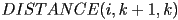and the second of these sub-paths has a length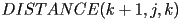. So, we simply write thatis the minimum ofand the sum ofwith. We perform this analysis on every pair of verticesand, and we successfully populatefor every pairand.

After repeating this process for everyfromto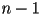, then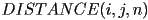will give us the minimum length path from vertexto vertex.